# MA.7.NSO.1.1Export Print
Know and apply the Laws of Exponents to evaluate numerical expressions and generate equivalent numerical expressions, limited to whole-number exponents and rational number bases.

### Clarifications

Clarification 1: Instruction focuses on building the Laws of Exponents from specific examples. Refer to the K-12 Formulas (Appendix E) for the Laws of Exponents.

Clarification 2: Problems in the form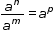must result in a whole-number value for p.

General Information
Subject Area: Mathematics (B.E.S.T.)
Strand: Number Sense and Operations
Status: State Board Approved

## Benchmark Instructional Guide

### Terms from the K-12 Glossary

• Base (of an exponent)
• Exponent (exponential form)
• Rational Number
• Whole Number

### Vertical Alignment

Previous Benchmarks

Next Benchmarks

### Purpose and Instructional Strategies

In previous courses, students evaluated positive rational numbers and integers with natural number exponents. In grade 7 accelerated, students use the Laws of Exponents to evaluate and generate numerical expressions, limited to whole-number exponents and rational number bases. Students extend their knowledge of the Laws of Exponents to generate equivalent algebraic expressions with integer exponents and monomial bases. In Algebra 1, students will use their knowledge of the Laws of Exponents to generate equivalent algebraic expressions with rational and variable exponents.
• Instruction includes allowing students to develop the Laws of Exponents based on patterns emerging from a series of examples related to each Law of Exponents (MTR.5.1).
• Develop understanding of the zero exponent by using multiplication to increase values and division to decrease values (MTR.5.1).
• For example, to show decreasing values, 5³ ÷ 5 = 5² and then 5² ÷ 5 = 5¹ (or 5) and then 5¹ ÷ 5 = 50 (or 5 ÷ 5 = 1).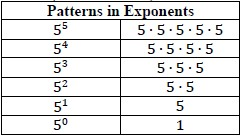• Full expansion of exponents, limited to whole number exponents and rational number bases, should be modeled to help develop these patterns.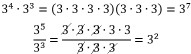• Students should develop fluency with and without the use of a calculator when evaluating numerical expressions involving the Laws of Exponents.
• Instruction includes cases where students must work backwards as well as cases where the value of a variable must be determined (MTR.3.1). Students should use relational thinking as well as algebraic thinking.
• For example, in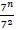= 343, what is the value of $n$? Students should ask themselves, “If I know that 343 is 7³, what value would $n$ need to be so that $n$ − 2 = 3?”
• For example, in (5²)$n$ = 510, what is the value of $n$? Students should ask themselves, “What power would 5² be raised to equal 510?”

### Common Misconceptions or Errors

• Students may incorrectly conclude that squaring a number means to multiply by 2. Likewise, cubing may be mistaken as multiplying by 3. Use length to show doubling and area of a square to show an exponent of 2. Use of two-dimensional and three-dimensional manipulatives (MTR.2.1) may also help to emphasize squares and cubes versus increasing length.
• When finding the product or quotient of powers, students may incorrectly multiply or divide the bases, rather than only manipulating the exponents. Use full expansion of the exponential expression (MTR.2.1) to develop the laws.
• Students may incorrectly invert the product of powers and power of a power laws, wanting to multiply in the first and add in the latter.

### Strategies to Support Tiered Instruction

• Instruction includes modeling the differences between doubling and squaring a value. Doubling a value would be represented by multiplying a given length by 2 whereas squaring a number would be represented by the area of a square with a given length.
• For example, students can be given the table below to show how the left column doubles a length whereas the right column squares a length.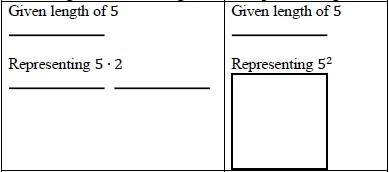• Instruction includes modeling the differences between tripling or cubing a value. Tripling a value would be represented by multiplying a given length by 3, whereas cubing a number would be represented by the volume of a cube with a given length.
• For example, students can be given the table below to show how the left column triples a length whereas the right column cubes a length.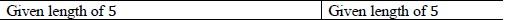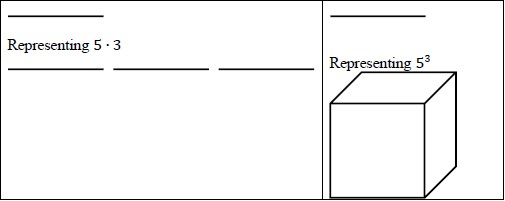• The teacher provides opportunities for students who incorrectly apply the Laws of Exponents when generating equivalent numerical expressions to use full expansion of exponential expression as an additional step to visually represent the emerging patterns.
• For example, the expression 4³ ⋅4² can be expanded to (4 ⋅ 4 ⋅ 4) ⋅ (4 ⋅ 4) which is equivalent to 4⋅4⋅4⋅4⋅4 or 45. This helps illustrate the product of powers Law.
• Teacher creates and posts an anchor chart with visual representations of the base of an exponent, exponent and any factor(s) then encourages students to utilize the anchor chart to assist in correct academic vocabulary when evaluating exponential expressions.
• For example, the teacher can highlight different parts of the expression 4³ ⋅4² in different colors to visualize the base, exponents and factors: 4³ · 4².
• Instruction includes co-creating a graphic organizer for each of the Laws of Exponents to include the name of the law, an original exponential expression, an equivalent expansion of the expression, the equivalent simplified expression, and a generalized rule for each of the laws.
• For example, a graphic organizer with some of the Laws is shown.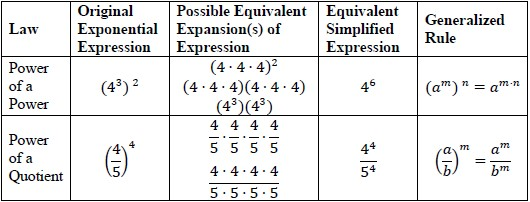Complete the table by using numeric examples to develop a rule for each of the situations given. Then confirm or adjust your hypothesis as one of the Laws of Exponents.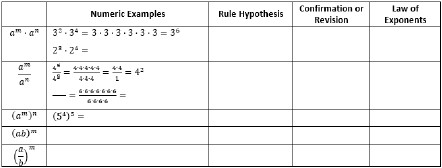### Instructional Items

Instructional Item 1
Generate an equivalent expression.
($\frac{\text{1}}{\text{2}}$)2 · ($\frac{\text{1}}{\text{2}}$)3

Instructional Item 2

Evaluate the following expression.
(($14$)2)3 · ($14$)3

Instructional Item 3
Evaluate the following expression.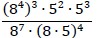*The strategies, tasks and items included in the B1G-M are examples and should not be considered comprehensive.

## Related Courses

This benchmark is part of these courses.
1205040: M/J Grade 7 Mathematics (Specifically in versions: 2014 - 2015, 2015 - 2022, 2022 and beyond (current))
1205050: M/J Accelerated Mathematics Grade 7 (Specifically in versions: 2014 - 2015, 2015 - 2020, 2020 - 2022, 2022 and beyond (current))
1204000: M/J Foundational Skills in Mathematics 6-8 (Specifically in versions: 2014 - 2015, 2015 - 2022, 2022 and beyond (current))
7812020: Access M/J Grade 7 Mathematics (Specifically in versions: 2014 - 2015, 2015 - 2018, 2018 - 2019, 2019 - 2022, 2022 and beyond (current))

## Related Access Points

Alternate version of this benchmark for students with significant cognitive disabilities.
MA.7.NSO.1.AP.1: Use properties of whole number exponents to produce equivalent expressions.

## Related Resources

Vetted resources educators can use to teach the concepts and skills in this benchmark.

## Original Student Tutorial

Applying the Pythagorean Theorem to Solve Mathematical and Real-World problems:

Apply the Pythagorean Theorem to solve mathematical and real-rorld problems in this interactive tutorial.

Type: Original Student Tutorial

## Tutorial

Powers of Zero:

Students will learn that non-zero numbers to the zero power equal one. They will also learn that zero to any positive exponent equals zero.

Type: Tutorial

## Original Student Tutorials Mathematics - Grades 6-8

Applying the Pythagorean Theorem to Solve Mathematical and Real-World problems:

Apply the Pythagorean Theorem to solve mathematical and real-rorld problems in this interactive tutorial.

## Student Resources

Vetted resources students can use to learn the concepts and skills in this benchmark.

## Original Student Tutorial

Applying the Pythagorean Theorem to Solve Mathematical and Real-World problems:

Apply the Pythagorean Theorem to solve mathematical and real-rorld problems in this interactive tutorial.

Type: Original Student Tutorial

## Tutorial

Powers of Zero:

Students will learn that non-zero numbers to the zero power equal one. They will also learn that zero to any positive exponent equals zero.

Type: Tutorial

## Parent Resources

Vetted resources caregivers can use to help students learn the concepts and skills in this benchmark.